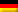At inlet and outlet boiling temperature ϑbD = 80,3 °C

For the specific condensation enthalpy of the overhead product, approximately that of benzene is used (p. 110):

ΔhB = 394000 J/kg

Required heat flow (p. 238):

Φ = qmD · ΔhB = 0,258 kg/s . 394000 J/kg = 101652 W

K value according to table (p. 235): K = 800 W/(m² – K)

Mean logarithmic temperature difference (p. 237):

Required exchange area (p. 239):

Dieser Beitrag ist auch verfügbar auf:Deutsch (German)